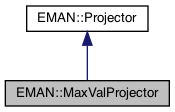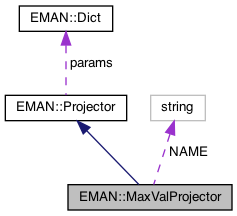EMAN2
EMAN::MaxValProjector Class Reference

Real-space projection which computes the maximum value along each line projection rather than a sum. More...

`#include <projector.h>`

Inheritance diagram for EMAN::MaxValProjector:[legend]
Collaboration diagram for EMAN::MaxValProjector:[legend]

## Public Member Functions

TypeDict get_param_types () const
Get processor parameter information in a dictionary. More...

EMDataproject3d (EMData *image) const
Project an 3D image into a 2D image. More...

EMDatabackproject3d (EMData *image) const
Back-project a 2D image into a 3D image. More...

string get_name () const
Get the projector's name. More...

string get_desc () constPublic Member Functions inherited from EMAN::Projector
virtual ~Projector ()

virtual Dict get_params () const
Get the projector parameters in a key/value dictionary. More...

void set_params (const Dict &new_params)
Set the projector parameters using a key/value dictionary. More...

## Static Public Member Functions

static ProjectorNEW ()

## Static Public Attributes

static const string NAME = "maxval"Protected Attributes inherited from EMAN::Projector
Dict params

## Detailed Description

Real-space projection which computes the maximum value along each line projection rather than a sum.

Parameters
 Transform object used for projection

Definition at line 300 of file projector.h.

## ◆ backproject3d()

 EMData * MaxValProjector::backproject3d ( EMData * image ) const
virtual

Back-project a 2D image into a 3D image.

Returns
A 3D image from the backprojection.

Implements EMAN::Projector.

Definition at line 2136 of file projector.cpp.

2137{
2138 // no implementation yet
2139 EMData *ret = new EMData();
2140 return ret;
2141}
EMData stores an image's data and defines core image processing routines.
Definition: emdata.h:82

## ◆ get_desc()

 string EMAN::MaxValProjector::get_desc ( ) const
inlinevirtual

Implements EMAN::Projector.

Definition at line 319 of file projector.h.

320 {
321 return "Real-space projection which computes the maximum value along each line projection rather than the sum";
322 }

## ◆ get_name()

 string EMAN::MaxValProjector::get_name ( ) const
inlinevirtual

Get the projector's name.

Each projector is indentified by unique name.

Returns
The projector's name.

Implements EMAN::Projector.

Definition at line 314 of file projector.h.

315 {
316 return NAME;
317 }
static const string NAME
Definition: projector.h:329

References NAME.

## ◆ get_param_types()

 TypeDict EMAN::MaxValProjector::get_param_types ( ) const
inlinevirtual

Get processor parameter information in a dictionary.

Each parameter has one record in the dictionary. Each record contains its name, data-type, and description.

Returns
A dictionary containing the parameter info.

Reimplemented from EMAN::Projector.

Definition at line 303 of file projector.h.

304 {
305 TypeDict d;
306 d.put("transform", EMObject::TRANSFORM, "Transform object used for projection");
307 return d;
308 }

References EMAN::TypeDict::put(), and EMAN::EMObject::TRANSFORM.

## ◆ NEW()

 static Projector * EMAN::MaxValProjector::NEW ( )
inlinestatic

Definition at line 324 of file projector.h.

325 {
326 return new MaxValProjector();
327 }

## ◆ project3d()

 EMData * MaxValProjector::project3d ( EMData * image ) const
virtual

Project an 3D image into a 2D image.

Returns
A 2D image from the projection.

A "fix" for the segmentation fault when calling initmodel.py with standard projector. We'll look into this and make a real fix. – Grant Tang

Implements EMAN::Projector.

Definition at line 944 of file projector.cpp.

945{
946 Transform* t3d = params["transform"];
947 if ( t3d == NULL ) throw NullPointerException("The transform object containing the angles(required for projection), was not specified");
948// Dict p = t3d->get_rotation();
949 if ( image->get_ndim() == 3 )
950 {
951
952 int nx = image->get_xsize();
953 int ny = image->get_ysize();
954 int nz = image->get_zsize();
955
956// Transform3D r(Transform3D::EMAN, az, alt, phi);
957 Transform r = t3d->inverse(); // The inverse is taken here because we are rotating the coordinate system, not the image
958 int xy = nx * ny;
959
960 EMData *proj = new EMData();
961 proj->set_size(nx, ny, 1);
962
963 Vec3i offset(nx/2,ny/2,nz/2);
964
965 float *sdata = image->get_data();
966 float *ddata = proj->get_data();
967 for (int k = -nz / 2; k < nz - nz / 2; k++) {
968 int l = 0;
969 for (int j = -ny / 2; j < ny - ny / 2; j++) {
970 ddata[l]=0;
971 for (int i = -nx / 2; i < nx - nx / 2; i++,l++) {
972
973 Vec3f coord(i,j,k);
974 Vec3f soln = r*coord;
975 soln += offset;
976
980// printf(" ");
981
982 float x2 = soln;
983 float y2 = soln;
984 float z2 = soln;
985
986 float x = (float)Util::fast_floor(x2);
987 float y = (float)Util::fast_floor(y2);
988 float z = (float)Util::fast_floor(z2);
989
990 float t = x2 - x;
991 float u = y2 - y;
992 float v = z2 - z;
993
994 size_t ii = (size_t) ((size_t)x + (size_t)y * nx + (size_t)z * xy);
995//
996 if (x2 < 0 || y2 < 0 || z2 < 0 ) continue;
997 if (x2 > (nx-1) || y2 > (ny-1) || z2 > (nz-1) ) continue;
998
999 if (x2 < (nx - 1) && y2 < (ny - 1) && z2 < (nz - 1)) {
1000 ddata[l] = Util::get_max(ddata[l],
1001 Util::trilinear_interpolate(sdata[ii], sdata[ii + 1], sdata[ii + nx],
1002 sdata[ii + nx + 1], sdata[ii + xy], sdata[ii + xy + 1], sdata[ii + xy + nx],
1003 sdata[ii + xy + nx + 1], t, u, v));
1004 }
1005 else if ( x2 == (nx - 1) && y2 == (ny - 1) && z2 == (nz - 1) ) {
1006 ddata[l] = Util::get_max(ddata[l],sdata[ii]);
1007 }
1008 else if ( x2 == (nx - 1) && y2 == (ny - 1) ) {
1009 ddata[l] = Util::get_max(ddata[l],Util::linear_interpolate(sdata[ii], sdata[ii + xy],v));
1010 }
1011 else if ( x2 == (nx - 1) && z2 == (nz - 1) ) {
1012 ddata[l] = Util::get_max(ddata[l],Util::linear_interpolate(sdata[ii], sdata[ii + nx],u));
1013 }
1014 else if ( y2 == (ny - 1) && z2 == (nz - 1) ) {
1015 ddata[l] = Util::get_max(ddata[l],Util::linear_interpolate(sdata[ii], sdata[ii + 1],t));
1016 }
1017 else if ( x2 == (nx - 1) ) {
1018 ddata[l] = Util::get_max(ddata[l],Util::bilinear_interpolate(sdata[ii], sdata[ii + nx], sdata[ii + xy], sdata[ii + xy + nx],u,v));
1019 }
1020 else if ( y2 == (ny - 1) ) {
1021 ddata[l] = Util::get_max(ddata[l],Util::bilinear_interpolate(sdata[ii], sdata[ii + 1], sdata[ii + xy], sdata[ii + xy + 1],t,v));
1022 }
1023 else if ( z2 == (nz - 1) ) {
1024 ddata[l] = Util::get_max(ddata[l],Util::bilinear_interpolate(sdata[ii], sdata[ii + 1], sdata[ii + nx], sdata[ii + nx + 1],t,u));
1025 }
1026 }
1027 }
1028 }
1029 proj->update();
1030 proj->set_attr("xform.projection",t3d);
1031 proj->set_attr("apix_x",(float)image->get_attr("apix_x"));
1032 proj->set_attr("apix_y",(float)image->get_attr("apix_y"));
1033 proj->set_attr("apix_z",(float)image->get_attr("apix_z"));
1034
1035 if(t3d) {delete t3d; t3d=0;}
1036 return proj;
1037 }
1038 else if ( image->get_ndim() == 2 ) {
1039
1040 Transform r = t3d->inverse(); // The inverse is taken here because we are rotating the coordinate system, not the image
1041
1042 int nx = image->get_xsize();
1043 int ny = image->get_ysize();
1044
1045 EMData *proj = new EMData();
1046 proj->set_size(nx, 1, 1);
1047 proj->to_zero();
1048
1049 float *sdata = image->get_data();
1050 float *ddata = proj->get_data();
1051
1052 Vec2f offset(nx/2,ny/2);
1053 for (int j = -ny / 2; j < ny - ny / 2; j++) { // j represents a column of pixels in the direction of the angle
1054 int l = 0;
1055 for (int i = -nx / 2; i < nx - nx / 2; i++,l++) {
1056
1057 Vec2f coord(i,j);
1058 Vec2f soln = r*coord;
1059 soln += offset;
1060
1061 float x2 = soln;
1062 float y2 = soln;
1063
1064 float x = (float)Util::fast_floor(x2);
1065 float y = (float)Util::fast_floor(y2);
1066
1067 int ii = (int) (x + y * nx);
1068 float u = x2 - x;
1069 float v = y2 - y;
1070
1071 if (x2 < 0 || y2 < 0 ) continue;
1072 if (x2 > (nx-1) || y2 > (ny-1) ) continue;
1073
1074 if ( x2 < (nx - 1) && y2 < (ny - 1) ) {
1075 ddata[l] = Util::get_max(ddata[l],Util::bilinear_interpolate(sdata[ii], sdata[ii + 1], sdata[ii + nx],sdata[ii + nx + 1], u, v));
1076 }
1077 else if (x2 == (nx-1) && y2 == (ny-1) ) {
1078 ddata[l] = Util::get_max(ddata[l],sdata[ii]);
1079 }
1080 else if (x2 == (nx-1) ) {
1081 ddata[l] = Util::get_max(ddata[l],Util::linear_interpolate(sdata[ii],sdata[ii + nx], v));
1082 }
1083 else if (y2 == (ny-1) ) {
1084 ddata[l] = Util::get_max(ddata[l],Util::linear_interpolate(sdata[ii],sdata[ii + 1], u));
1085 }
1086 }
1087 }
1088 proj->set_attr("xform.projection",t3d);
1089 proj->update();
1090 if(t3d) {delete t3d; t3d=0;}
1091 return proj;
1092 }
1093 else throw ImageDimensionException("Standard projection works only for 2D and 3D images");
1094}
A Transform object is a somewhat specialized object designed specifically for EMAN2/Sparx storage of ...
Definition: transform.h:75
Transform inverse() const
Get the inverse of this transformation matrix.
Definition: transform.cpp:1327
static float linear_interpolate(float p1, float p2, float t)
Calculate linear interpolation.
Definition: util.h:518
static int fast_floor(float x)
A fast way to calculate a floor, which is largest integral value not greater than argument.
Definition: util.h:874
static float trilinear_interpolate(float p1, float p2, float p3, float p4, float p5, float p6, float p7, float p8, float t, float u, float v)
Calculate trilinear interpolation.
Definition: util.h:619
static float bilinear_interpolate(float p1, float p2, float p3, float p4, float t, float u)
Calculate bilinear interpolation.
Definition: util.h:543
static float get_max(float f1, float f2)
Get the maximum of 2 numbers.
Definition: util.h:998
The Vec2 is precisely the same as Vec3 except it works exclusively in 2D Note there are convenient ty...
Definition: vec3.h:710
#define ImageDimensionException(desc)
Definition: exception.h:166
#define NullPointerException(desc)
Definition: exception.h:241
#define y(i, j)
Definition: projector.cpp:1516
#define x(i)
Definition: projector.cpp:1517

## ◆ NAME

 const string MaxValProjector::NAME = "maxval"
static

Definition at line 329 of file projector.h.

Referenced by get_name().

The documentation for this class was generated from the following files: